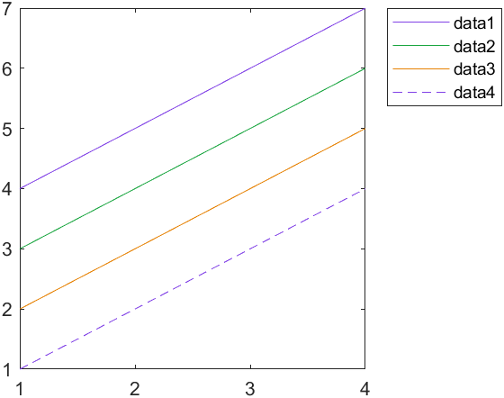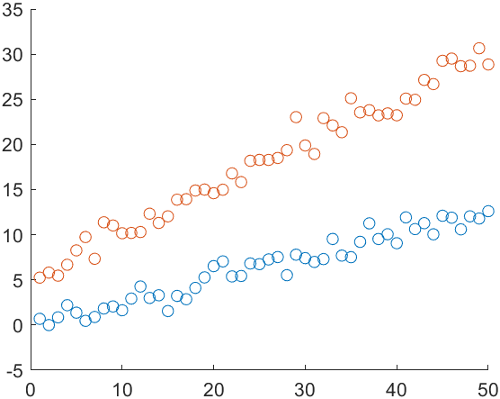## Control Automatic Selection of Colors and Line Styles in Plots

When you plot multiple data sets together in the same axes, MATLAB® helps you to distinguish your data sets by varying their appearance. For example, when you plot multiple lines, MATLAB assigns each line a color from a set of colors according to their order of creation. If you create more lines than there are colors, MATLAB repeats the cycle of colors starting with the first color. You can change this behavior:

This topic demonstrates how to configure line and scatter plots, but the same concepts for controlling the cycling of colors (and possibly line styles) apply to many other plots, including `bar`, `area`, and `stem` plots. All of the examples in this topic set the axes properties after plotting. This sequence of steps is important, because most plotting functions reset many of the axes properties.

Note

If you want to specify a color or a line style for a specific item in a plot, see Specify Plot Colors and Specify Line and Marker Appearance in Plots.

### Specify Different Sets of Colors and Line Styles

The colors MATLAB selects come from the axes `ColorOrder` property, which contains a three-column matrix of colors specified as RGB triplets. An RGB triplet is three-element vector containing the intensities of the red, green, and blue components of a color. The intensities must be in the range [0, 1].

If you plot multiple lines, the first line uses the first color in the `ColorOrder` matrix, the second line uses the second color, and so on. Eventually, the colors repeat if the plot has more lines than rows in the matrix. This code creates several line plots that use the default color order. The first line is the top-most line. Because the default `ColorOrder` matrix has seven rows, the colors repeat after the seventh line.

```plot([9 10 11 12]) hold on plot([8 9 10 11]) plot([7 8 9 10]) plot([6 7 8 9]) plot([5 6 7 8]) plot([4 5 6 7]) plot([3 4 5 6]) plot([2 3 4 5]) plot([1 2 3 4]) hold off legend("Location","northeastoutside")```You can change the colors in either of two ways:

• Set the `ColorOrder` property of the axes to a new matrix of RGB triplets.

• Call the `colororder` function. This function accepts RGB triplets, color names, such as `"red"`, and hexadecimal color codes (since R2019b). It also accepts any of several predefined palette names, such as `"gem"`, `"reef"` or `"meadow"` (since R2023b).

Create a new matrix containing the RGB triplets for red, green, and blue. Then set the `ColorOrder` property to that matrix. The plot updates immediately with the new colors.

```mycolors = [1 0 0; 0 1 0; 0 0 1]; ax = gca; ax.ColorOrder = mycolors;```MATLAB also cycles through different line styles in addition to colors. By default, there is only one line style (a solid line). To specify additional line styles, set the `LineStyleOrder` property of the axes. For example, this code specifies three line styles. The updated plot cycles through all the colors with one line style before displaying the next line style.

```mylinestyles = ["-"; "--"; "-o"]; ax.LineStyleOrder = mylinestyles;```### Specify Cycling Order of Colors and Line Styles

Since R2023a

When you use multiple colors and line styles, you can specify whether the plot cycles through all line styles before, after, or at the same time as the colors by setting the `LineStyleCyclingMethod` property. This property can have one of three values:

• `"aftercolor"` — Cycle through the line styles after the colors. This value is the default.

• `"beforecolor"` — Cycle through the line styles before the colors.

• `"withcolor"` — Cycle through the line styles with the colors.

Plot four lines. Set the `LineStyleOrder` property to three line styles, and set the `ColorOrder` property to three colors by passing an array of three hexadecimal color codes to the `colororder` function. Then add a legend.

```% Plot four lines plot([4 5 6 7]) hold on plot([3 4 5 6]) plot([2 3 4 5]) plot([1 2 3 4]) hold off % Set the line style order and color order ax = gca; ax.LineStyleOrder = ["-"; "--"; "-o"]; colororder(["#8040E6";"#1AA640";"#E68000"]) legend("Location","northeastoutside")```By default, the plot cycles through all the colors with the first (solid) line style before it displays the next (dashed) line style. Because there are three colors, only two of the line styles are used in the four-line plot.

If you want more of your lines to be distinct in both color and line style, use the `"withcolor"` option and specify an equal number of colors and line styles. For example, change the `LineStyleCylingMethod` of the preceding plot to `"withcolor"`. The updated plot cycles through the colors and the line styles at the same time.

`ax.LineStyleCyclingMethod = "withcolor";`### Group Your Data by Color or Line Style

Since R2020a

To group related lines (or other plot objects) together visually, set the `SeriesIndex` property of each object that you want to receive the same visual treatment to the same number. The `SeriesIndex` property indexes into the `ColorOrder` and `LineStyleOrder` arrays according to the value of the `LineStyleCyclingMethod` property. Thus, different objects with the same `SeriesIndex` value use the same color (and line style, if applicable).

For example, plot two sets of 50 scattered points.

```x = 1:50; meas1 = 0.25*x + randn(1,50); scat1 = scatter(x,meas1); hold on meas2 = 0.5*x + randn(1,50) + 5; scat2 = scatter(x,meas2);```Calculate a fit line for each set of points using the `polyfit` and `polyval` functions. Then add each fit line to the plot.

```% Calculate fit lines for each set of measurements p1 = polyfit(x,meas1,1); y1_fit = polyval(p1,x); p2 = polyfit(x,meas2,1); y2_fit = polyval(p2,x); % Plot fit lines fitline1 = plot(x,y1_fit); fitline2 = plot(x,y2_fit); hold off```The scatter plots and fit lines are all different colors. The reason is that MATLAB selects a new color from the `ColorOrder` matrix for each new plot you add to the axes.

Match the color of each fit line with its associated scatter plot. Set the `SeriesIndex` property of each line to the same value that the associated scatter plot has.

```fitline1.SeriesIndex = scat1.SeriesIndex; fitline2.SeriesIndex = scat2.SeriesIndex;```This relationship persists even if you change the colors in the `ColorOrder` matrix. For example, change the colors to purple and green by calling the `colororder` function.

`colororder([0.5 0.25 0.90; 0.10 0.65 0.25])`